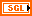# Floating-Point Numbers

## LabVIEW 2018 Help

Edition Date: March 2018
Part Number: 371361R-01
View Product InfoLabVIEW 2016 HelpLabVIEW 2017 HelpLabVIEW 2018 HelpLabVIEW 2019 HelpLabVIEW 2020 Help

Floating-point numbers in LabVIEW conform to the ANSI/IEEE Standard 754-1985. Not all real numbers can be represented in the ANSI/IEEE standard floating-point numbers. Because of this, comparisons using floating-point numbers may yield results you do not expect because of rounding errors. To avoid inaccurate results, you can round floating-point numbers to integers. For example, if you want the result of a calculation to contain two digits of precision, multiply the floating-point number by 100 and then round the product to an integer before you complete the calculation. You also can check to see whether two floating-point numbers are close to each other instead of equal to each other. For example, if the absolute value of the difference of two floating-point numbers is smaller than a defined tolerance, assume the numbers are equal.

Refer to the Numeric Data Types Table for more information about numeric data type bits, digits, and range. There are three types of floating-point numbers.Single-precision (SGL)—Single-precision, floating-point numbers have 32-bit IEEE single-precision format. Use single-precision, floating-point numbers when memory savings are important and you will not overflow the range of the numbers.Double-precision (DBL)—Double-precision, floating-point numbers have 64-bit IEEE double-precision format. Double-precision is the default format for numeric objects. For most situations, use double-precision, floating-point numbers.Extended-precision (EXT)—When you save extended-precision numbers to disk, LabVIEW stores them in a platform-independent 128-bit format. In memory, the size and precision vary depending on the platform. Use extended-precision, floating-point numbers only when necessary. The performance of extended-precision arithmetic vary among platforms.

Data Acquisition VIs often return arrays of floating-point numbers.